﻿ 船用燃机液体耦合式间冷器的稳态运行优化
«上一篇文章快速检索 高级检索

 哈尔滨工程大学学报2019, Vol. 40Issue (4): 767-773  DOI: 10.11990/jheu.2018010010

### 引用本文JI Niankun, WANG Zhitao, LI Shuying. Optimization of steady-state operation of fluid-coupling type intercooler applied to marine gas turbines[J]. Journal of Harbin Engineering University, 2019, 40(4), 767-773. DOI: 10.11990/jheu.201801001.### 文章历史

Optimization of steady-state operation of fluid-coupling type intercooler applied to marine gas turbines
JI Niankun , WANG Zhitao, LI Shuying
College of Power and Energy Engineering, Harbin Engineering University, Harbin 150001, China
Abstract: To optimize the steady-state operation of a fluid coupling-type intercooler applied to marine gas turbines, a dimensionless parameter called equivalent intercooler effectiveness was defined and its feasibility to use a single parameter to characterize the effect of intercooler on gas turbine performance was proven.Thus, the coupling between heat exchangers and that between the intercooler and gas turbine was weakened.A mathematical model was built to calculate the off-design condition of the fluid coupling-type intercooler.Calculation results under off-design condition indicated that both temperature and pressure drops on the air side of the intercooler have a linear negative correlation with equivalent intercooler effectiveness, while the operating energy consumption has a significant nonlinear positive correlation with equivalent intercooler effectiveness.Furthermore, taking the maximum contribution of the intercooler to gas turbine performance and minimum energy consumption of the intercooler itself as objective function, we established a multi-objective optimization model of the intercooler operating under steady state.A stratified sequencing method based on variable step search was designed to solve the multi-objective optimization problem.The simulation results showed that this method could perform fast convergence to a global optimal unique solution.
Keywords: gas turbine    fluid coupling-type intercooler    off-design condition    multi-objective optimization    variable step search    stratified sequencing method    steady-state operation

1 液体耦合式间冷器物理模型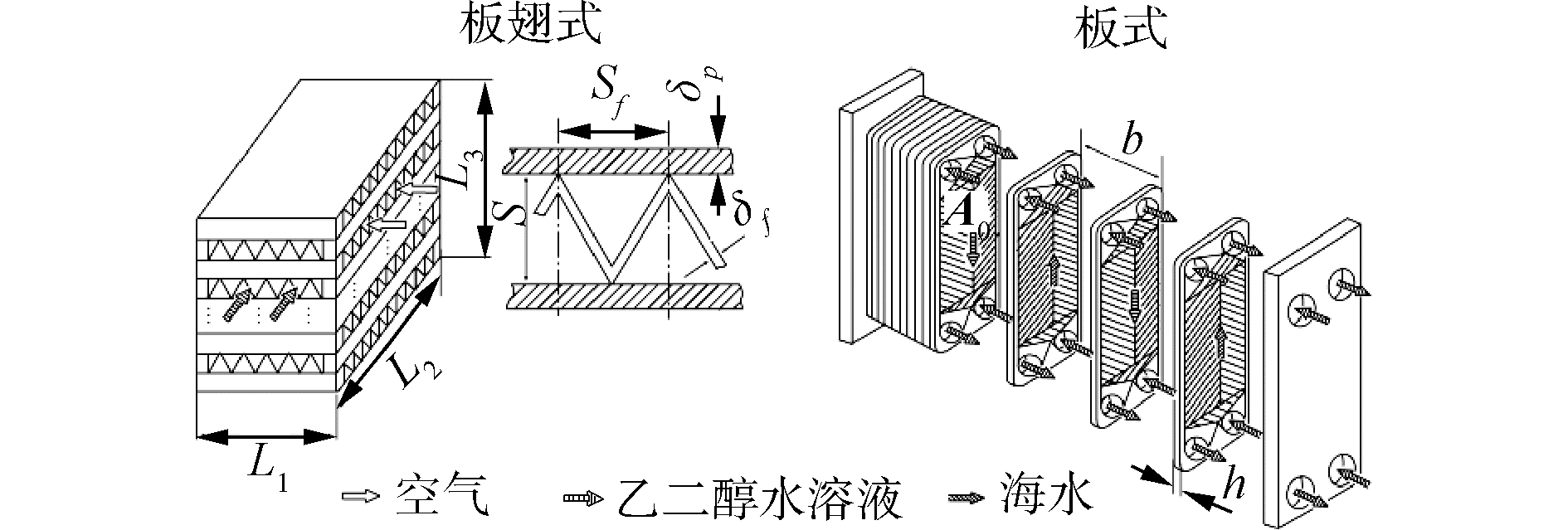Download: 图 1 液体耦合式间冷器物理模型 Fig. 1 Physical model of fluid coupling type intercooler表 1 机上间冷器结构参数 Table 1 Structure parameters of on-engine intercooler表 2 机外间冷器结构参数 Table 2 Structure parameters of off-engine intercooler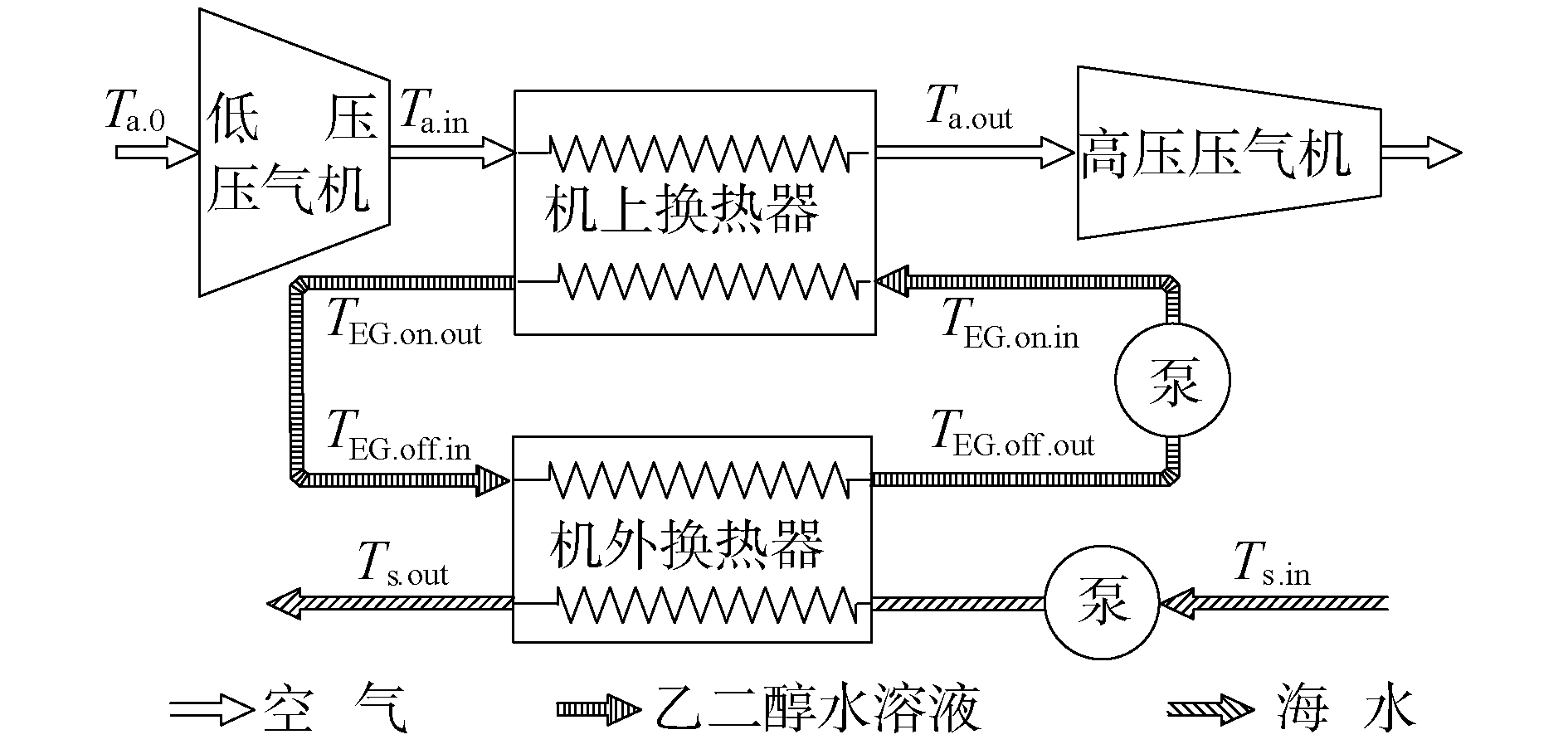Download: 图 2 间冷器装机示意 Fig. 2 Schematic of intercooler installed on gas turbine

 ${T_{{\rm{EG}}{\rm{.on}}{\rm{.in}}}} = {T_{{\rm{EG}}{\rm{.off}}{\rm{.out}}}}$ (1)
 ${T_{{\rm{EG}}{\rm{.on}}{\rm{.out}}}} = {T_{{\rm{EG}}{\rm{.off}}{\rm{.in}}}}$ (2)

1) 忽略管路中的换热和压力损失，认为系统的换热和压力损失全部由换热器芯体产生；

2) 忽略海面的海—气温差，认为机上间冷器空气侧入口温度与海水表面温度相等[11-13]

3) 忽略间冷器冷凝，将环境大气简化为干空气处理。

2 稳态变工况计算 2.1 等效间冷度定义及可行性分析

 ${\sigma _{{\rm{on}}}} = \frac{{{T_{{\rm{a}}{\rm{.in}}}} - {T_{{\rm{a}}{\rm{.out}}}}}}{{{T_{{\rm{a}}{\rm{.in}}}} - {T_{{\rm{EG}}{\rm{.on}}{\rm{.in}}}}}}$ (3)
 ${\sigma _{{\rm{off}}}} = \frac{{{T_{{\rm{EG}}{\rm{.off}}{\rm{.in}}}} - {T_{{\rm{EG}}{\rm{.off}}{\rm{.out}}}}}}{{{T_{{\rm{EG}}{\rm{.off}}{\rm{.in}}}} - {T_{{\rm{s}}{\rm{.in}}}}}}$ (4)

 ${\sigma _{{\rm{eq}}}} = \frac{{{T_{{\rm{a,in}}}} - {T_{{\rm{a,out}}}}}}{{{T_{{\rm{a,in}}}} - {T_{{\rm{s,in}}}}}}$ (5)

 $\begin{array}{*{20}{c}} {\frac{1}{{{\sigma _{{\rm{eq}}}}}} = \frac{1}{{{\sigma _{{\rm{on}}}} \cdot {\sigma _{{\rm{off}}}}}} + }\\ {\frac{{{T_{{\rm{a}}{\rm{.in}}}} - {T_{{\rm{EG}}{\rm{.off}}{\rm{.in}}}}}}{{{\sigma _{{\rm{on}}}}\left( {{T_{{\rm{a}}{\rm{.in}}}} - {T_{{\rm{EG}}{\rm{.off}}{\rm{.in}}}}} \right) + {\sigma _{{\rm{on}}}} \cdot {\sigma _{{\rm{off}}}}\left( {{T_{{\rm{EG}}{\rm{.off}}{\rm{.in}}}} - {T_{{\rm{s}}{\rm{.in}}}}} \right)}} \cdot }\\ {\left( {1 - \frac{1}{{{\sigma _{{\rm{off}}}}}}} \right)} \end{array}$ (6)

 $\frac{1}{{{\sigma _{{\rm{eq}}}}}} < \frac{1}{{{\sigma _{{\rm{on}}}} \cdot {\sigma _{{\rm{off}}}}}}且\frac{1}{{{\sigma _{{\rm{eq}}}}}} > \frac{1}{{{\sigma _{{\rm{on}}}} \cdot {\sigma _{{\rm{off}}}}}} + \frac{1}{{{\sigma _{{\rm{on}}}}}}\left( {1 - \frac{1}{{{\sigma _{{\rm{off}}}}}}} \right)。$

σonσoffσeq三者之间的不等式关系为：

 ${\sigma _{{\rm{on}}}} \cdot {\sigma _{{\rm{off}}}} < {\sigma _{{\rm{eq}}}} < {\sigma _{{\rm{on}}}}$ (7)

 ${T_{{\rm{a}}{\rm{.in}}}} = {T_{a.0}}\left[ {1 + \frac{{{\pi _{{\rm{CL}}}}^{\frac{{k - 1}}{k}} - 1}}{{{n_{{\rm{CL}}}}}}} \right]$ (8)

 ${T_{{\rm{s}}{\rm{.in}}}} = {T_{{\rm{a}}.0}}$ (9)

 $a\left( {{\pi _{{\rm{CL}}}},{n_{{\rm{CL}}}}} \right) = 1 + \frac{{{\pi _{{\rm{CL}}}}^{\frac{{k - 1}}{k}} - 1}}{{{n_{{\rm{CL}}}}}}$ (10)

 $\beta = \frac{{{T_{{\rm{a}}.{\rm{out}}}}}}{{{T_{{\rm{a}}.0}}}}$ (11)

 ${\sigma _{{\rm{eq}}}} = \frac{{\alpha - \beta }}{{\alpha - 1}}$ (12)

2.2 稳态变工况耦合换热模型

2.2.1 机上换热器计算

 $\begin{array}{*{20}{c}} {{q_{{\rm{EG}}{\rm{.on}}}}{C_{{\rm{pEG}}{\rm{.on}}}}\left( {{T_{{\rm{EG}}{\rm{.on}}{\rm{.out}}}} - {T_{{\rm{EG}}{\rm{.on}}{\rm{.in}}}}} \right) = }\\ {{q_{\rm{a}}}C{q_{\rm{a}}}\left( {{T_{{\rm{a}}{\rm{.in}}}} - {T_{{\rm{a}}{\rm{.out}}}}} \right)} \end{array}$ (13)

 $\Delta {P_{\rm{a}}} = g_{{\rm{m}}{\rm{.a}}}^2 \cdot {\mathit{\boldsymbol{v}}_{{\rm{a}}{\rm{.in}}}}\left[ {\left( {\frac{{{\mathit{\boldsymbol{v}}_{{\rm{a}}{\rm{.out}}}}}}{{{\mathit{\boldsymbol{v}}_{{\rm{a}}{\rm{.in}}}}}} - 1} \right) + 2\frac{{{f_a} \cdot {L_1} \cdot {\mathit{\boldsymbol{v}}_{{\rm{a}}{\rm{.m}}}}}}{{{d_{{\rm{ea}}}} \cdot {\mathit{\boldsymbol{v}}_{{\rm{a}}{\rm{.in}}}}}}} \right]$ (14)

 $\begin{array}{*{20}{c}} {\Delta {p_{{\rm{EG}}{\rm{.on}}}} = g_{{\rm{m}}{\rm{.EG}}{\rm{.on}}}^2 \cdot {\mathit{\boldsymbol{v}}_{{\rm{EG}}{\rm{.on}}{\rm{.in}}}}\left[ {\left( {\frac{{{\mathit{\boldsymbol{v}}_{{\rm{EG}}{\rm{.on}}{\rm{.out}}}}}}{{{\mathit{\boldsymbol{v}}_{{\rm{EG}}{\rm{.on}}{\rm{.in}}}}}} - 1} \right) + } \right.}\\ {\left. {2\frac{{{f_{{\rm{EG}}{\rm{.on}}}} \cdot {L_2} \cdot {\mathit{\boldsymbol{v}}_{{\rm{EG}}{\rm{.on}}{\rm{.m}}}}}}{{{d_{{\rm{e}}{\rm{.EG}}{\rm{.on}}}} \cdot {\mathit{\boldsymbol{v}}_{{\rm{EG}}{\rm{.on}}{\rm{.in}}}}}}} \right]} \end{array}$ (15)
2.2.2 机外换热器计算

 ${q_{{\rm{EG}}{\rm{.off}}}} = {q_{{\rm{EG}}{\rm{.on}}}}$ (16)

 $\begin{array}{*{20}{c}} {{q_{\rm{s}}}{C_{{\rm{ps}}}}\left( {{T_{{\rm{s}}{\rm{.out}}}} - {T_{{\rm{s}}{\rm{.in}}}}} \right) = }\\ {{q_{{\rm{EG}}{\rm{.off}}}}{C_{{\rm{pEG}}{\rm{.off}}}}\left( {{T_{{\rm{EG}}{\rm{.off}}{\rm{.in}}}} - {T_{{\rm{EG}}{\rm{.off}}{\rm{.out}}}}} \right)} \end{array}$ (17)

 ${V_s} = \frac{{{q_{{\rm{EG}}{\rm{.off}}}}}}{{{\rho _{{\rm{EG}}}} \cdot {n_1} \cdot {A_{{\rm{s}}{\rm{.EG}}}}}}$ (18)

 ${K_{{\rm{off}}}} = \frac{{{q_{\rm{s}}}}}{{{\rho _{\rm{s}}} \cdot {n_2} \cdot {A_{\rm{s}}}}}$ (19)

 ${K_{{\rm{off}}}} = {\left( {\frac{1}{{{\alpha _{{\rm{EG}}}}}} + \frac{1}{{{\alpha _{\rm{s}}}}} + {R_0}} \right)^{ - 1}}$ (20)

 ${\alpha _{{\rm{EG}}{\rm{.off}}}} = \frac{{{N_{{\rm{u}}{\rm{.EG}}{\rm{.off}}}} \cdot {\lambda _{{\rm{EG}}{\rm{.off}}}}}}{{d{e_{{\rm{EG}}{\rm{.off}}}}}}$ (21)
 ${\alpha _{\rm{s}}} = \frac{{{N_{{\rm{u}}{\rm{.s}}}} \cdot {\lambda _{\rm{s}}}}}{{{d_{{\rm{es}}}}}}$ (22)

 ${{K'}_{{\rm{off}}}} = \frac{\phi }{{{A_{{\rm{off}}}} \cdot \Delta {t_{{\rm{m}}{\rm{.off}}}}}}$ (23)

 $\Delta {P_{{\rm{EG}}{\rm{.off}}}} = {N_{{\rm{u}}{\rm{.EG}}{\rm{.off}}}} \cdot {\rho _{{\rm{EG}}{\rm{.off}}}} \cdot V_{{\rm{EG}}{\rm{.off}}}^2$ (24)

 $\Delta {P_{\rm{s}}} = {E_{{\rm{u}}{\rm{.s}}}} \cdot {\rho _{\rm{s}}} \cdot V_{\rm{s}}^2$ (25)
2.2.3 泵功耗的计算

 $\Delta {P_{{\rm{EG}}}} = {P_{{\rm{EG}}{\rm{.on}}}} + {P_{{\rm{EG}}{\rm{.off}}}}$ (26)

 ${P_{{\rm{b}}{\rm{.EG}}}} = \frac{{{q_{{\rm{EC}}}} \cdot \Delta {P_{{\rm{EG}}}}}}{{{\rho _{{\rm{EG}}}}}}$ (27)

 ${P_{{\rm{b}}{\rm{.s}}}} = \frac{{{q_{\rm{s}}} \cdot \Delta {P_{\rm{s}}}}}{{{\rho _{\rm{s}}}}}$ (28)
2.3 稳态变工况算例及特性分析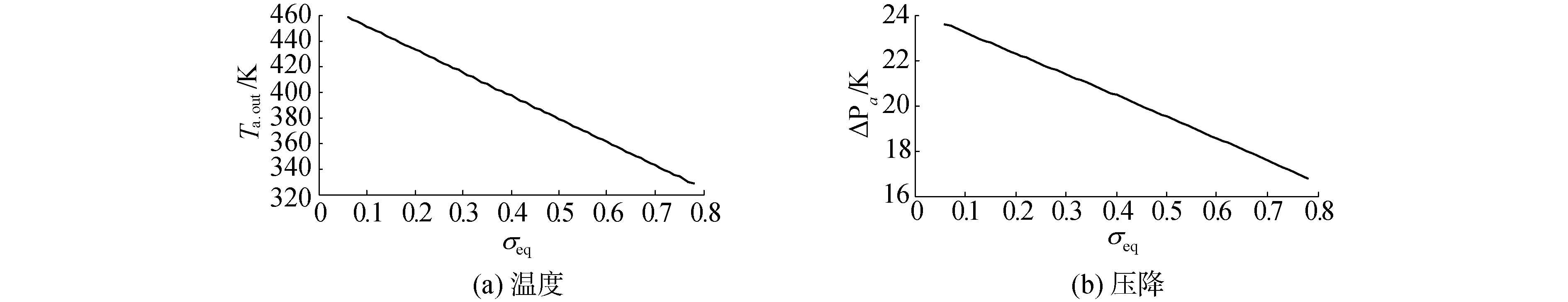Download: 图 3 空气侧出口参数随等效间冷度的变化曲线 Fig. 3 Airside parameters versus equivalent intercooler effectiveness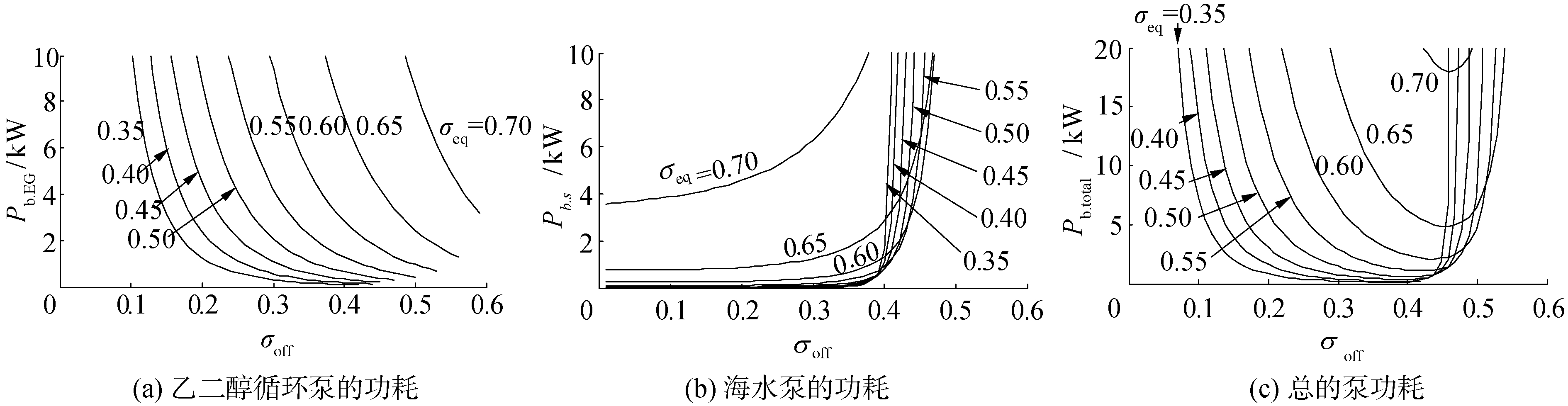Download: 图 4 泵功耗随机外换热效率的变化曲线 Fig. 4 Pump power versus off-engine intercooler effectiveness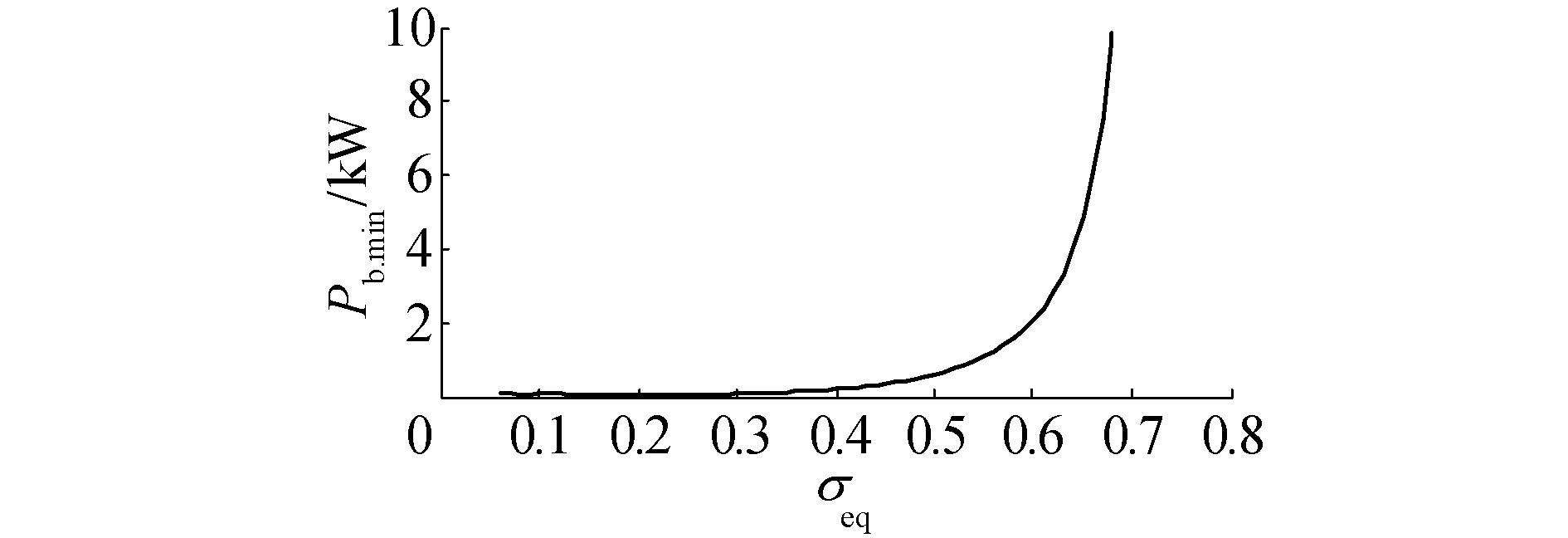Download: 图 5 Pb.min随σeq的变化曲线 Fig. 5 Minimum total pump power versus equivalent intercooler effectiveness

3 稳态运行优化 3.1 多目标优化问题描述

 $\left\{ \begin{array}{l} \max \;\;\;{\sigma _{{\rm{eq}}}} = {f_1}\left( \mathit{\boldsymbol{x}} \right)\\ \min \;\;\;{P_{{\rm{b}}{\rm{.total}}}} = {f_2}\left( \mathit{\boldsymbol{x}} \right)\\ {\rm{s}}.\;{\rm{t}}.\;\;\;\;{g_i}\left( \mathit{\boldsymbol{x}} \right) \le 0,i = 1,2, \cdots ,\mathit{\boldsymbol{m}}\\ \;\;\;\;\;\;\;\;{P_{{\rm{b}}{\rm{.EG}}}}\left( \mathit{\boldsymbol{x}} \right) \le {P_{{\rm{b}}{\rm{.EG}}{\rm{.d}}}}\\ \;\;\;\;\;\;\;\;{P_{{\rm{b}}{\rm{.s}}}}\left( \mathit{\boldsymbol{x}} \right) \le {P_{{\rm{b}}{\rm{.s}}{\rm{.d}}}} \end{array} \right.$ (29)

3.2 基于变步长搜索的分层序列法求解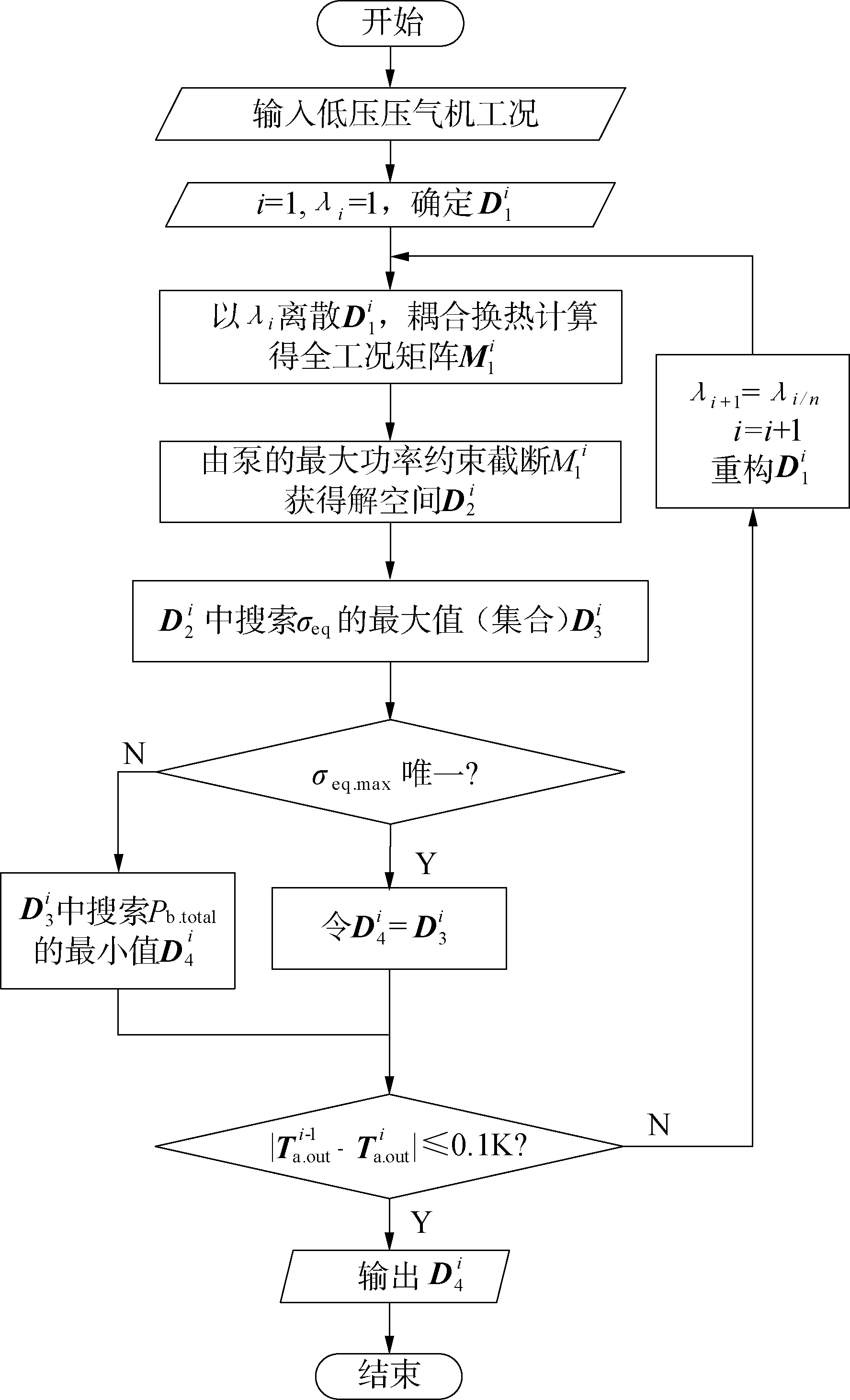Download: 图 6 优化流程 Fig. 6 Optimization flow chart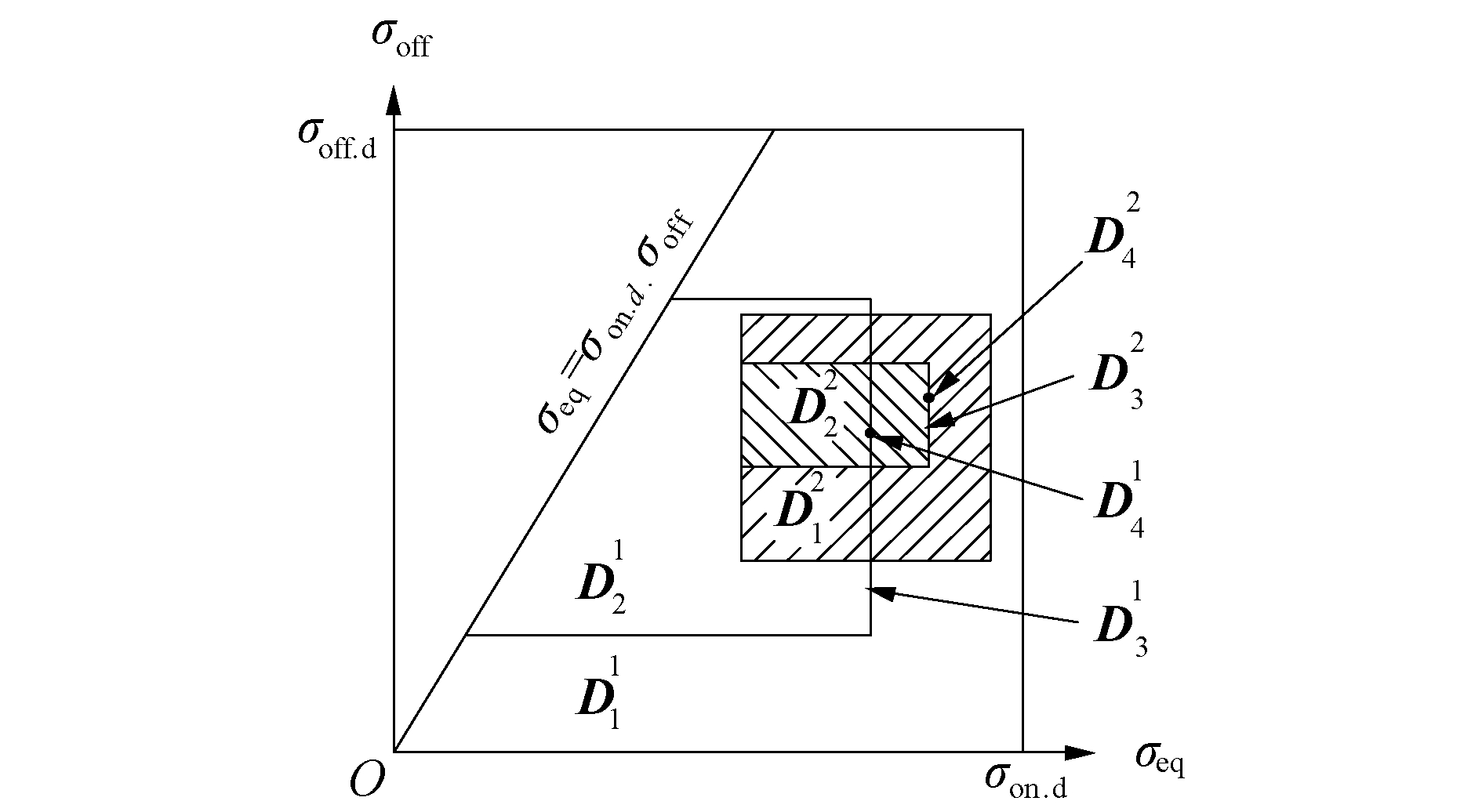Download: 图 7 优化算法的解域 Fig. 7 Solving domain of optimization algorithm

1) 置迭代计数器i = 1, 由决策向量x的取值范围确定初始搜索空间D1i(图 7中为一梯形)，记${\mathit{\boldsymbol{D}}_1}^i = \left\{ {\mathit{\boldsymbol{x}}\left| 0 \right. \le {\sigma _{{\rm{off}}}} \le {\sigma _{{\rm{off}}{\rm{.d}}}}} \right\} \cap \{ \mathit{\boldsymbol{x}}\left| {{\sigma _{{\rm{on}}{\rm{.d}}}}} \right. \cdot {\sigma _{{\rm{off}}}} \le {\sigma _{{\rm{eq}}}} \le {\sigma _{{\rm{on}}{\rm{.d}}}}\}$σeqσoff的初始步长均取为λi

2) 针对空间D1i中以步长λi离散后的各点实施间冷器耦合换热计算，获得优化目标函数(σeqPb.total)值以及约束条件(Pb.EGPb.s)值，与决策变量(σeqσoff)一起构成间冷器全工况运行数据M1i

3) 由约束条件Pb.EG(x)≤Pb.EG.dPb.s(x)≤Pb.s.d截断M1i，确定满足约束条件的解空间D2i(图 7中为一梯形)，记${\mathit{\boldsymbol{D}}_2}{^i} = \left\{ {\mathit{\boldsymbol{x}}|{P_{{\rm{b}}{\rm{.EG}}}}\left(\mathit{\boldsymbol{x}} \right) \le {P_{{\rm{b}}{\rm{.EG}}{\rm{.d}}}}} \right\} \cap \left\{ {\mathit{\boldsymbol{x}}|{P_{{\rm{b}}{\rm{.s}}}}\left(\mathit{\boldsymbol{x}} \right) \le {P_{{\rm{b}}{\rm{.s}}{\rm{.d}}}}} \right\} \cap \mathit{\boldsymbol{D}}{_1}^i$

4) 在空间D2i中搜索主要目标σeq的最大值σeq.max，获得主要目标函数最优值集合D3i，记D3i = {x|σeq = σeq.max}∩ D2i

5) 在D3i中搜索次要目标Pb.total的最小值Pb.min，获得次要目标函数最优值集合D4i(图 7中为一点)，记D4i = {x|Pb.total = Pb.min}∩ D3i

6) 若Ta.outi-1-Ta.outi＞0.1 K，则以D4i为中心，以2λi为边长重设搜索空间D1i+1(正方形)，并重设搜索步长λi+1 = λi/nn为步长收缩因子。置迭代计数器i = i+1, 转2)。

Ta.outi-1-Ta.outi＞0.1 K，则输出D 4i

3.3 稳态运行优化算例及分析表 3 低压压气机工作参数 Table 3 Operating parameters of low-pressure compressor表 4 间冷器运行优化结果 Table 4 Optimization results of intercooler operation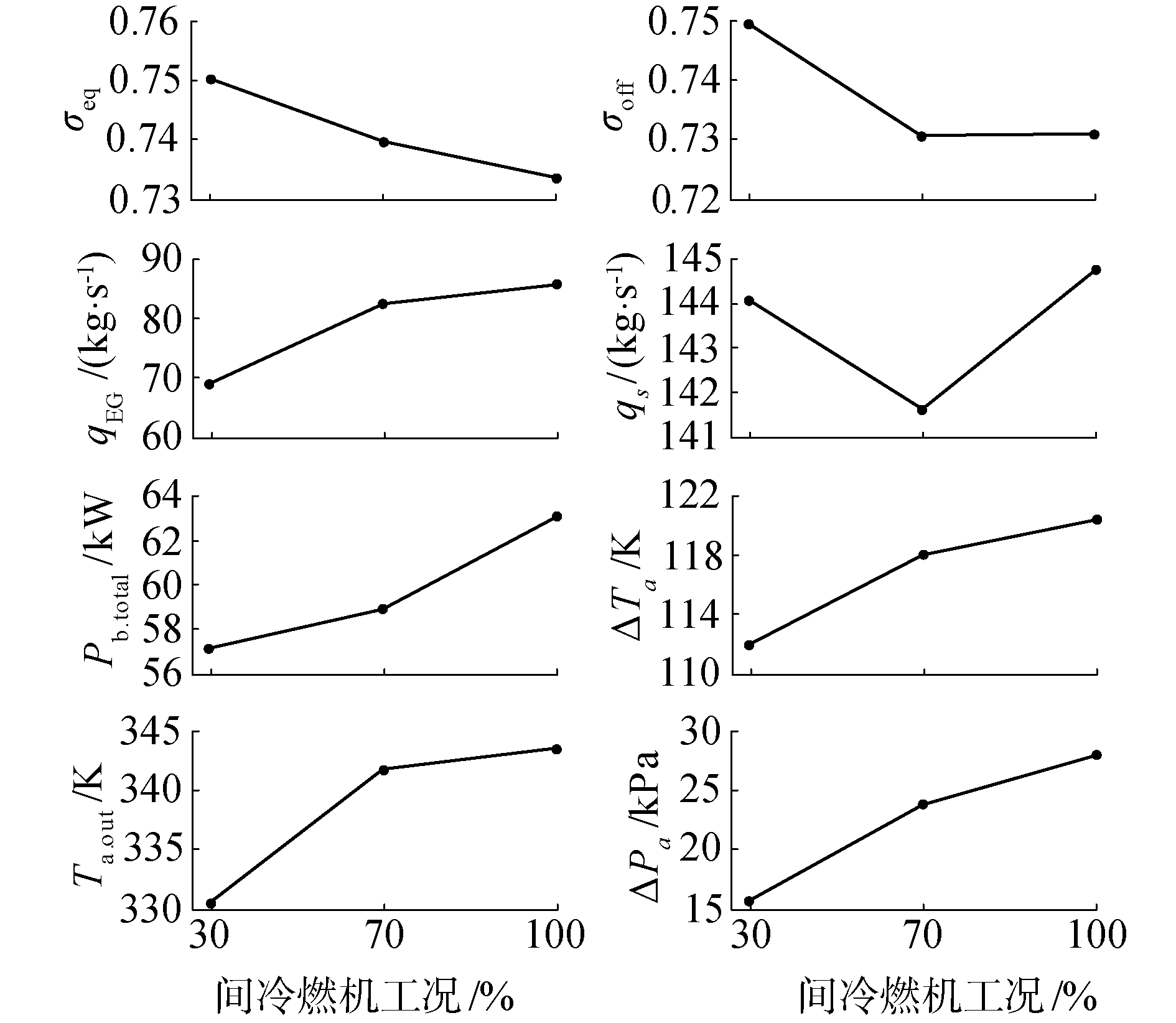Download: 图 8 间冷器参数随燃机工况的变化曲线 Fig. 8 Operating parameters of intercooler versus working conditions of gas turbine

4 结论

1) 等效间冷度可作为表征间冷器对燃机性能影响的单一参数，其定义可在仿真中弱化间冷器内部及间冷器与燃机之间的耦合。

2) 推导机上、机外换热器换热效率和等效间冷度三者间的关系，并建立液体耦合式间冷器的稳态变工况计算模型。该模型迭代变量少，循环次数少，计算效率高。仿真结果揭示了间冷器运行状态对提高燃机性能和降低间冷器能耗的矛盾性。

3) 建立了液体耦合式间冷器稳态运行的多目标优化模型，并为此设计了一种基于变步长搜索的分层序列求解方法。仿真结果表明该方法收敛快，精度高。

4) 间冷器运行优化的建模及求解方法，可为进一步开展间冷器燃机整体性能优化以及间冷燃机控制策略的选择提供一定参考。

  余建祖. 换热器原理与设计[M]. 北京: 北京航空航天大学出版社, 2006: 1-7. (0)  SHEPARD S B, BOWEN T L, CHIPRICH J M. Design and development of the WR-21 intercooled recuperated (ICR) marine gas turbine[J]. Journal of engineering for gas turbines and power, 1995, 117(3): 557-562. DOI:10.1115/1.2814131 (0)  孙爱军, 肖娜新, 马力. WR-21舰船用燃气轮机的设计特点[J]. 航空发动机, 2010, 36(4): 49-52, 31. SUN Aijun, XIAO Naxin, MA Li. Design characteristics of WR-21 marine gas turbine[J]. Aeroengine, 2010, 36(4): 49-52, 31. DOI:10.3969/j.issn.1672-3147.2010.04.013 (0)  MCCARTHY S J, SCOTT I. The WR-21 intercooled recuperated gas turbine engine: operation and integration into the royal navy type 45 destroyer power system[C]//ASME Turbo Expo 2002: Power for Land, Sea, and Air. Amsterdam, The Netherlands, 2002: 977-984. (0)  董威, 高鹏, 郑培英, 等. 舰船燃气轮机间冷器优化设计与性能分析[J]. 航空发动机, 2011, 37(3): 22-25. DONG Wei, GAO Peng, ZHENG Peiying, et al. Optimal design and performance analysis of marine gas turbine intercooler[J]. Aeroengine, 2011, 37(3): 22-25. DOI:10.3969/j.issn.1672-3147.2011.03.008 (0)  肖鑫, 屈卫东. 基于改进Alopex算法的燃机中冷器优化设计[J]. 计算机仿真, 2013, 30(9): 318-321. XIAO Xin, QU Weidong. Improved Alopex algorithm on the optimization design of gas turbine intercooler[J]. Computer simulation, 2013, 30(9): 318-321. DOI:10.3969/j.issn.1006-9348.2013.09.074 (0)  ZHAO N B, WEN X Y, LI S Y. Dynamic time-delay characteristics and structural optimization design of marine gas turbine intercooler[J]. Mathematical problems in engineering, 2014, 2014: 701843. (0)  张善科.船用间冷循环燃气轮机性能仿真研究[D].北京: 中国舰船研究院, 2012: 24-36. ZHANG Shanke. Simulation research on performance of marine intercooled cycle gas turbine[D]. Beijing: China Ship Research Institute, 2012: 24-36. http://cdmd.cnki.com.cn/Article/CDMD-10217-1011020556.htm (0)  张杨.燃气轮机间冷器性能分析及优化设计[D].哈尔滨: 哈尔滨工程大学, 2014: 43-57. ZHANG Yang. Optimal design and performance analysis of heat transfer for gas turbine intercooler[D]. Harbin: Harbin Engineering University, 2014: 43-57. http://cdmd.cnki.com.cn/Article/CDMD-10217-1017244894.htm (0)  程宝华, 李先瑞. 板式换热器及换热装置技术应用手册[M]. 北京: 中国建筑工业出版社, 2005. (0)  LIN Ailian, LIANG Jianyin, GU Dejun, et al. On the relationship between convection intensity of south china sea summer monsoon and air-sea temperature difference in the tropical oceans[J]. Acta oceanologica sinica, 2004, 23(2): 267-278. (0)  熊学军. 中国近海海洋-物理海洋与海洋气象[M]. 北京: 海洋出版社, 2012. (0)  CHOI H, ZHANG Yuanhang. Monthly variation of sea-air temperature differences in the Korean coast[J]. Journal of oceanography, 2004, 61(5): 359-367. (0)  赵士杭. 燃气轮机循环与变工况性能[M]. 北京: 清华大学出版社, 1993: 19-23. (0)  张秀利, 梁迎春, 董申. 多目标结构模糊优化的分层序列法[J]. 机械设计与制造, 1999(5): 44-45. ZHANG Xiuli, LIANG Yingchun, DONG Shen. Lexicographic method for fuzzy multiobjective optimization of structures[J]. Machinery design & manufacture, 1999(5): 44-45. DOI:10.3969/j.issn.1001-3997.1999.05.019 (0)